Select Page

In classful IP addressing, there are five classes which are given below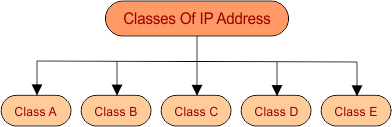In each class, two hosts IP are reserved

• First host-IP in the network which is zero (i.e. for class A (1.0.0.0) represents to that network and cannot be assign to any host in the network.
• And last IP in the network (i.e. for class A (126.255.255.255) used for broadcasting.

In each class, Some Leading Bits are reserved For Class Identification

• For class A, The first one leading bit is always fix to “0”
• For class B, The first two leading bits are always fixed to “10”
• For class C, The first three leading bits are always fixed to “110”
• For class D, The first four leading bits are always fixed to “1110”
• For class E, The first four leading bits are always fixed to “1111”

Look at the following diagram for batter understandingNumber of networks and number of hosts in each class can be calculated through the following formula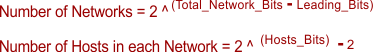Important To find Subnet mask of each class, Replace all Host octets to Zero and Network Octets to 255.

### Class A

• First octet (8-bits) represent network ID and remaining 3-octet (24-bits) represents the host ID. The first 1 leading bit of network ID in Class A is always (0) and remaining 7-bits represent network ID.
• Format: 0NNNNNNN.HHHHHHHH.HHHHHHHH.HHHHHHHH (in binary)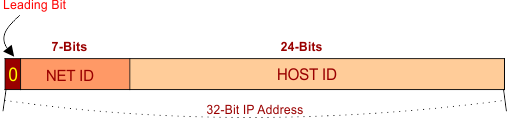• Start to End IP Address = (0.0.0.0 to 127.255.255.255)
• Value of first octet = 0 – 127
• Possible network ID’s = (27) = 128

Important Note: In class A, Network ID Zero (i.e. 0.0.0.0)  is reserved for default network and Network ID 127 (i.e. 127.0.0.0) is reserved for loopback (used for software testing). So, the  remaining 126 (1-126) networks ID’s are used in class A.

• Possible Hosts = (224-2) = 16777214
• Total number of possible IP addresses = (231) = 2,147,483,648 (because “1 out of 32” bit is leading bit)
• First IP address = 0.0.0.0
• First Host address = 0.0.0.1
• Last IP address = 127.255.255.255
• Last Host address = 127.255.255.254

Example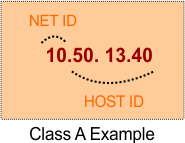Note: Class A Network is used for large size companies because it has large number of hosts.

### Class B

First two octets (16-bits) represent network ID and remaining 2-octets (16-bits) represents the host ID. The first two leading bit of network ID in Class B are always (10) and remaining 14-bits represent network ID.

• Format: 10NNNNNN. NNNNNNNN.HHHHHHHH.HHHHHHHH (in binary)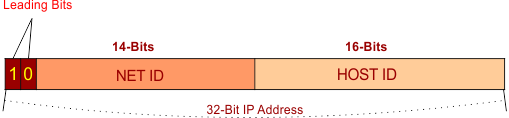• Value of first octet = (10000000 – 10111111) = 128 – 191
• Possible network ID = (214) = 16384
• Possible Hosts = (216-2) = 65534
• Possible Total number of IP addresses = (230) = (2 out of 32 bit is leading bit)
• First IP address = 128.0.0.0
• First Host address = 128.0.0.1
• Last IP address = 191.255.255.255
• Last Host address = 191.255.255.254

Example:

###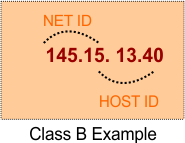Note: Class B Network is used for medium size companies because it has small number of hosts as compare to class A.

### Class C

First three octets (24-bits) represent network ID and remaining 1-octet (8-bits) represents the host ID. The first three leading bit of network ID in Class B are always (110) and remaining 21-bits  represent network ID.

• Format: 110NNNNN. NNNNNNNN. NNNNNNNN.HHHHHHHH (in binary)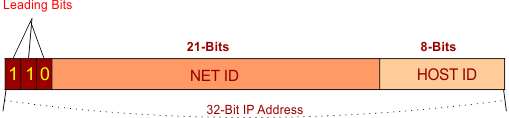• Value of first octet = (11000000 – 11011111) = 192 – 223
• Possible network ID = (221) = 2097152
• Possible Hosts = (28-2) = 254
• Possible Total number of IP addresses = (229) = (3 out of 32 bit is leading bit)
• First Host address = 192.0.0.1
• Last IP address = 223.255.255.255
• Last Host address = 223.255.255.254

Example: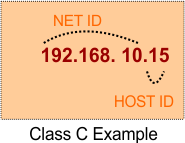Note: Class C Network is used for small size companies because it has small number of hosts as compare to class A and B.

### Class D

Class D is used for multicasts. Multicasting is used to pass the copies of datagram to selected groups of hosts instead of individual host. Class D is slightly different class from first three classes.

The first four leading bit of network ID in Class D are always (1110) and remaining 28-bits  represent group of computers where the multicast message will be passed.

• Format: 1110mmmm.mmmmmmmm.mmmmmmmm.mmmmmmmm (in binary)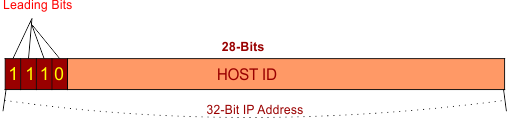• Value of first octet = (11100000 – 11101111) = 224 – 247
• Possible Total number of IP addresses = (228) = (4 out of 32 bit is leading bit)
• First IP address = 224.0.0.0
• First Host address = 224.0.0.1
• Last IP address = 239.255.255.255
• Last Host address = 223.255.255.254
• Subnet Mask, Network ID and Host ID are not defined in Class D

Example### Class E

Class E is used for future Experimental purpose only. It is mostly used in research and development fields.  The first four leading bit of network ID in Class E are always (1111)

• Format: 1111rrrr. rrrrrrrr. rrrrrrrr. rrrrrrrr (in binary)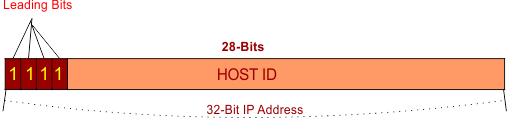• Value of first octet = (11110000 – 11111111) = 248 – 255)
• Possible Total number of IP addresses = (228) = (4 out of 32 bit is leading bit)
• First IP address = 240.0.0.0
• First Host address = 240.0.0.1
• Last IP address = 255.255.255.255
• Last Host address = 223.255.255.254
• Subnet Mask, Network ID and Host ID are not defined in Class E

Example: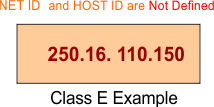### Important Points

• In a single network, All hosts holds the same network ID but different Host ID.
• Two hosts in different networks having different network ID but may have the same host ID.
• Last Network ID (i.e. 255.255 in Class B) with Last IP Address of that class (i.e. 255.255 in class B) is always a limited broadcast address of that class. Limited broadcast address of any class will always = 255.255.255.2555.
• Any Network ID (i.e. 135.115 in class B) with its Last IP Address  (i.e. 255.255 in class B) is always a direct broadcast address of that class. direct broadcast address of Network ID 135.115 is = 135.115.255.2555.
Help Other’s By Sharing…